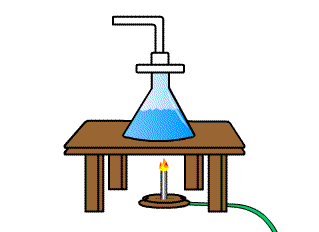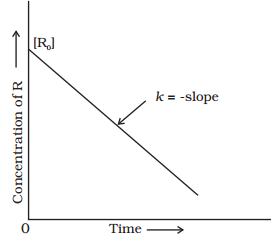Click to Chat

1800-1023-196

+91-120-4616500

CART 0

• 0

MY CART (5)

Use Coupon: CART20 and get 20% off on all online Study Material

ITEM
DETAILS
MRP
DISCOUNT
FINAL PRICE
Total Price: Rs.

There are no items in this cart.
Continue ShoppingZero Order Reactions

Table of Content

Examples of Zero Order Reactions

Characteristics of Zero Order Reaction

Half-Time or Half-Life Period of a Zero Order Reaction

Related ResourcesA reaction is said to be of zero order if its rate is independent of the concentration of the reactants, i.e., the rate is proportional to the zeroth power of the concentration of the reactants.

For the reaction

A → products

to be of zero order,d[A] = -kdt

Integrating both sides
[A] = – k t + C.....(i)
where, C is the constant of integration

At t = 0, the concentration of the reactant A = [A]0, where [A]0 is initial concentration of the reactant.
Substituting in equation (i)
[R]0 = –k × 0 + C
[R]0 = C
Substituting the value of C in the equation (i)
[A] = -kt + [A]0 ….. (ii)

Comparing equation (ii) with equation of a straight line.

y = mx + c

If we plot [A] against t, we get a straight line with slope = –k and intercept equal to [A]0.Some photochemical reaction and a few heterogeneous reactions are zero-order reactants. Such reactions are not common.

You can refer to the following video for the zero order reactions

Examples of Zero Order Reactions:

Photochemical reaction between hydrogen and chlorine:

H2(g) Cl2(g)    2HCl(g)

This photochemical reaction is zero-order reaction. The reaction is studied by placing H2 and Cl2 gases over water. The rate of reaction is studied by nothing the rate at which water rises in the vessel due to dissociation of HCl formed. the rate of rise of water is the same as the rate of disappearance of H2 and Cl2, i.e., the concentration of the gases phase will not change with time, although the quantities will change.

Decomposition of N2O on hot platinum surface:

N2O →  N2  +  1/2 O2

Rate  [N2O]0 =  k[N2O]0 = k

d[N2 O]/dt = k

Decomposition of NH3 in presence of molybdenum or tungsten is a zero-order reaction.

2NH3   N2 + 3H2

The surface of the catalyst is almost completely covered by NH3 molecules. The adsorption of gas on the surface cannot change by increasing the pressure or concentration of NH3. Thus, the concentration of gas phase remains constant although the product is formed. Therefore, this reaction zero order kinetics.

Other examples of zero order are:

Iodations of acetone in presence of H+ ions.

CH3COCH + I2    ICH2COCH3 + HI

The rate equation of this reaction does not include [I2] factor, i.e.,

-dx/dt = k[CH3 COCH3 ][H+]

Characteristics of Zero Order Reaction

The concentration of reactant decreases linearly with time.[A]t  =  [A]0 - kt

The time required for the reaction to be complete, i.e., time at which [A] is zero.
tcompletion = [A]0/k = (Initial concentration)/(Rate constant)

The units of k are mol L-1 time-1.

Half-Time or Half-Life Period of a Zero Order Reaction

The half-time of a reaction is defined as the time required to reduce the concentration of the reactant to half of its initial value. It is denoted by the symbol t1/2. Thus,

For zero order reaction, we know that

[A] = -kt + [A]0

at  half life of reaction,

t = t½ & [A] = [A]o/2

SoQuestion 1:

Rate of zero order reaction.

a. depends on concentration of reactants.

b. depends on concentration of products.

c. depends on concentration of both reactant and product.

d. does not depend on concentrations.

Question 2:

Unit of rate constant for a zero order reaction is

a. mol L-1 time-2.

b. mol2 L-1 time-1.

c. mol L-1 time-1.

d. mol-1 L-1 time-1.

Question 3:

If we plot [A] against t, we get a straight line with slope =

a. rate constant

b. arrhenius constant

c. temperature coefficient

d. equilibrium constant

Question 4:

For zero order reaction d[A] =

a. -kdt

b.  -k[A]0

c.-k[A]0dt

d. -k[A]0Q.1
Q.2
Q.3
Q.4

d
c
a
a

Related Resources

Look here for Books of Chemistry for IIT JEE

You can also refer to Introduction to chemical kinetics

To read more, Buy study materials of Chemical Kinetics comprising study notes, revision notes, video lectures, previous year solved questions etc. Also browse for more study materials on Chemistry here.### Course Features

• 731 Video Lectures
• Revision Notes
• Previous Year Papers
• Mind Map
• Study Planner
• NCERT Solutions
• Discussion Forum
• Test paper with Video Solution